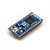## mbed VBUS voltage divider?

Looking at the mbed NXP LPC1768 Schematic one can see that the USB's 5V on VBUS is divided by a voltage divider R7 and R8 to provide the VBUS signal to the mbed interface IC at pin 58. A similar voltage divider for the LP1768 itself is in the reference design.

I find the dimensioning of these resistors puzzling. In both schematics these are 10k and 100k. The voltage ratio of the divider will be 100/(10+100)=0.91, i.e., not much reduction in voltage. I thought the voltage divider was there because the LPC1768's 5V tolerant pins are only 5V tolerant while supply voltage is applied.

Indeed the LPC1769/68/67/66/65/64/63 Product data sheet, section 15.1, reads:

#### Quote:

For systems where VDD can be 0 V and VBUS is directly applied to the VBUS pin, precautions must be taken to reduce the voltage to below 3.6 V. The maximum allowable voltage on the VBUS pin is 3.6 V. One method is to use a voltage divider to connect the VBUS pin to the VBUS on the USB connector.

The voltage divider ratio should be such that the VBUS pin will be greater than 0.7VDD to indicate a logic HIGH while below the 3.6 V allowable maximum voltage.

Use the following operating conditions:

VBUSmax = 5.25 V

VDD = 3.6 V

The voltage divider would need to provide a reduction of 3.6 V/5.25 V or 0.686.

The Application Note AN11392 ('Guidelines for full-speed USB on NXP's LPC microcontrollers') suggests 24k and 39k ohm resistors.

Did mbed miss the mark here, with the 10k and 100k?

If one accidentally draws too much power from the mbed, so the FPF2123 limits the current and the supply voltage drops, then the VBUS pin is fried by the USB voltage?

#### Question relating to:Rapid Prototyping for general microcontroller applications, Ethernet, USB and 32-bit ARM® Cortex™-M3 based designs

##### 3 years, 7 months ago.

I dont actually see the voltage divider in the LPC1768 reference design. It only shows in the IF schematic of the original LPC1768 mbed module.

The ''magic'' IF chip on the original LPC1768 module is an LPC2141 (or one of its familymembers with more memory eg LPC2148). The VBUS pin on these device has a max voltage rating of 5V25. It may be connected directly to the USB power pin (see datasheet). So this is different from the LPC1768 max voltage which would indeed need a voltage divider as you concluded.